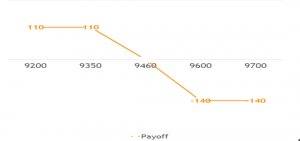Search

# Bearish Options Trading Strategies – How to Use Options in Bearish Market?

Bearish Options Trading Strategies Explained: If the Bulls express their view in the Options market by Buying Call Option, then the Bears express their view by Buying Put Option. In this article, we will try and understand the option strategies for the BEARISH Market.

“Call Options give the right to the Holder of the option the right (not the obligation) to buy the underlying asset on or before expiry, whereas the Put Option gives the holder the right (not the obligation) to sell the underlying asset on or before expiry!”

Option trading need not always be a game of large gains or nothing. There are various strategies that can be devised to express one’s view and take minimal risk and earn decent returns.

Bearish markets are those markets where the expectation of weakness in the price of the underlying asset is expected to continue. Let us try and understand few option trading strategies for Bearish markets, that have the potential to generate decent returns by taking moderate risks. Keep reading.

Here are two of the common bearish options trading strategies for beginners to make profits trading options:

Assumptions while using this strategy:

Bear Put Spread is a Two-legged strategy i.e. there are two options positions running simultaneously. There is an assumption of weakness in the market and the next level of supports is likely to be tested by the market.

Implementation of Bear Put Strategy

1. We have to buy One In the Money Put Option

2. Sell One Out of Money Put Option

The following are the assumptions under this strategy:

1. All the strikes belong to the same underlying Asset.

2. Both the options have the same expiry

3. And both the legs have the same number of contracts

Let us understand this strategy with the help of an example:

Spot Price of Nifty: 10050

The Following are the option legs traded:

1. An In the Money (ITM) Put Option = 10200 PE, premium Paid = 180 units

2. And, An out of Money (OTM) Put Option = 10000 PE, premium received = 60 units

Overall, this is a ‘Net Debit’ strategy. The P/L at the beginning of this strategy is -120 units(60-180)

Let us understand the payoff at various levels of expiry:

Case 1: Nifty upon expiry goes up to 10400, then:The total P/L (net payoff) in this case is -120 units (-180+60)

Case 2: If Nifty upon expiry goes up to 10300, then:The total P/L (net payoff) in this case is -120 units (-180+60)

Case 3: If Nifty upon expiry goes up to 10080, then:The total P/L (net payoff) in this case is 0 units (-60+60). This is also the BEP for this strategy

Case 4: If Nifty upon expiry goes to 10000, then:The total P/L (net payoff) in this case is 80units (20+60)

Case 5: If Nifty upon expiry goes to 9800, then:The total P/L (net payoff) in this case is 80 units (220-140)

## Payoff Using Bear Put Spread1. The Maximum and Minimum profit potential is capped in this strategy

2. The summation of maximum profit and loss is equal to the difference between two chosen strike prices

3. The Breakeven Point in this strategy is reached when the spot price is the difference between the higher strike price and ‘Net Debit’

Bear Call Spread is another Bearish Options trading strategy. This strategy also runs on the same assumption as the Bear Put Spread. There are two legs in this strategy and there is an expectation of weakness in the market. But unlike the Bear Put strategy, In the Bear Call strategy, we have two legs of Call options positions running simultaneously. And we can express our Bearish view by selling Call options and pocket the premium.

Implementation of this strategy

To execute this strategy, we need to:

1. Buy one Out of Money call option

2. Sell one In the Money call option

Both the Options should have the same expiry date and same underlying Asset

Let us understand this strategy with the help of an example. Assume, the sot price of Nifty is 9500

The following are the legs in this strategy:

1. Buy one Out of Money Call option of 9600 CE, premium paid: 60 units

2. Sell one In the Money Call option of 9350 CE, the premium received = 170 units

The net payoff at the time of entering the contract is 11o units (170-60). This is a Net Credit strategy

Case 1: If the Nifty Expires at 9700, then:The Net payoff using this strategy will be -140 units (40-180)

Case 2: If the Nifty Expires at 9600, then:The Net payoff using this strategy will be -140 units (-60-80)

Case 3: If the Nifty Expires at 9460, then:The Net payoff using this strategy will be 0 units (-60+60). This is also the point of Breakeven in this strategy.

Case 4: If the Nifty Expires at 9350, then:The Net payoff using this strategy will be 110units (-60+170)

Case 4: If the Nifty Expires at 9200, then:The Net payoff using this strategy will be 110 units (-60+170)

## Payoff using Bear Call Strategy